# Random Walks

A drunk man will find his way home, but a drunk bird may get lost forever.

Shizuo Kakutani

Our goal in this section is to prove the above statement. If your probability is rusty (or nonexistent) this review might be useful. We start by describing the behavior of our drunkards mathematically. Conceptually a random walk is exactly what it sounds like. Our drunkard starts at a "home" vertex, 0, and then choses at random a neighboring vertex to walk to next. We let X(n) denote the walkers position at time n. The drunkard returns home when X(n) = X(0). Frequently we can accurately calculate the probability that the walker returns home in n steps, and we denote this probability of return as q(n). As our walkers are allowed to retrace their steps we always have that q(2n) > 0, so this probability can't be what Prof. Kakutani was referring to. Before we continue there is another important fact to mention. If the walker or bird is moving on a finite graph then there's no way to get lost forever; there are simply not enough places to go.

## Activity 1

Try to come up with an example of a graph in which q(2n+1) = 0 for all natural numbers n. Similarly come up with a graph in which q(2n+1) > 0 for all large enough natural numbers. Do these properties depend on the starting vertex of the walker?

The key here is that the bird may get lost forever, that is the bird can only return home some finite number of times. After its last return home the bird then flies off never to return again. We want to get a grasp on the probability of this happening. For simplicity of notation we will assume that the bird never returns home at all. Don't be bothered by this; since the bird's past steps don't influence his future steps beyond giving his current position we can imagine him returning home any number of times (even 0) before this final flight.

For reasons more technical than we want to get into, returning home infinitely many times either always happens or never happens. What this means is that if you were able to run the entire walk in a finite amount of time, each time you ran the experiment you would get the same result in terms of this behavior. If the walker always return we say he is recurrent, and otherwise he is transient. Luckily there is a straight-forward criteria for checking recurrence: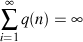If this is the case the walker is recurrent. Otherwise the walker is transient. You should think of this sum as being the expected number of times the walker returns home. For our purposes we will show that q(2n) is approximately n-r for some positive r. If r > 1 the sum is finite and so the walker is transient; otherwise it is infinite and the walker is recurrent.

## The Drunk Man

Continuing this discussion in full generality is going to get messy, so from now on we'll talk about specific graphs. We'll start with our drunkard in a very large (infinite) but boring town: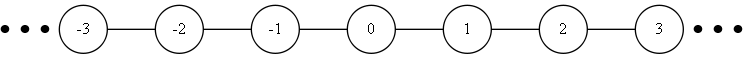This is just the one-dimensional number line of the integers, Z. Home is 0. At each step our walker will flip a fair coin. On a heads he moves to the right and on a tails to the left. To formalize this we speak of the transition probability, p(u, v), of the walker moving from v to u in one step. If we want to talk about the transition probabilities for n steps we write pn(u,v) instead. Note that q(n)=pn(0,0).

## Activity 2

Write down the one-step transition probabilities for the walk described above. To avoid making a really long list you might want to think about p(i,j) where i and j are arbitrary integers.

## Activity 3

Try to find a general formula for q(2n) on Z in terms of combinations. It might help to think about how many possible paths there are of length 2n, and under what conditions will such a path return to the origin at the last step. Once you have done this use Stirling's approximation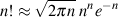to get an estimate of q(2n).

Plugging the result of the last activity into our test for recurrence we can see that on Z the drunkard will return home infinitely many times.

Now we move to a larger city Z2:Again the walker will start at the origin, and we will label vertices by integer coordinates. At each step the walker will move either up, down, left or right, each with probability 1/4. For a taste of what the walker's path might look below are sample walks of 50, 500, 5000 and 50000 steps. Notice how the walker doesn't stray too far from home.The argument used above works similarly in this case, though there is a bit more work involved in finding q(2n). However, there is a clever way to avoid this. We imagine two walkers in one-dimension, except that one of them moves in the directions (1,1) and (-1,-1) and the other in the directions (1,-1) and (-1,1). Let Y(n) and Z(n) denote their positions. The sum of their positions Y(n)+Z(n) defines another walk on Z2 corresponding to the dotted edges in this graph:## Activity 4

Check that X(n) has the same transition probabilities as the walk determined by (Y(n)+Z(n))/2. This means that we have decomposed our walk on Z2 into two copies of the walk on Z that we already understand.

Since these two new walkers move independently of each other on Z we have: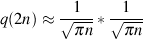Plugging this into the test for recurrence shows that the walk on Z2 is recurrent as well.

## The Drunk Bird

Now we can turn to our drunken bird. We now move to the three-dimensional graph Z3. Vertices in this graph correspond to triples of integers. Similarly, the bird has 6 possible directions to move in, each with probability 1/6. We call these directions N, E, S, W, U and D. We now need to do some accounting of the number of paths the bird can take.

In order for the bird to return in 2n steps, n of these steps most be in the up, N, or E directions. There are C(2n,n) ways to do this. Amongst these n steps i must go up, j must go N, and n-i-j must go E. There are C(n,i)C(n-i,j) ways to do this.

## Activity 5

Why are there C(n,i)C(n-i,j) ways to do this? It might help to think of this in terms of placing n identical balls into 3 urns labeled U, N, and E of capacities i, j, and n-i-j respectively.

Since i and j can vary up to the point where their sum is at most n, we have that the number of possible paths of 2n steps which return home is:If the square is confusing you, remember that we also need to pick where S, W, and D fall as well. Our big difficulty is the sum, however the function f(x,y,z)=x!y!z! under the constraint x+y+z=n is maximized when x, y and z are close to being equal (feel free to inspect this with a computer/calculator). This lets us get rid of the square. Also, there are 62n total configurations of our 6 step directions of length 2n, and combining these facts gives: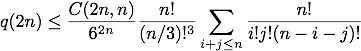Now we use a little combinatorial trick. The remaining sum is really just counting the number of ways to put n identical balls into 3 distinct buckets. Another way to count this is to note that each ball has 3 options as to where it can go, so there are 3n possible ways to do this. Now we just use this and apply Stirling's approximation (details omitted to spare the reader) again to get that: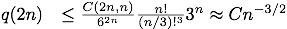Where C is some positive number that has no influence on recurrence. Applying our recurrence test now we see that the bird is transient, and so it will eventually leave home forever. Similar arguments can be used to show that the walker is transient in all higher dimensions.

Go Back